TitleCollege Algebra
Tutorial 10: Adding and Subtracting Rational ExpressionsLearning Objectives

 After completing this tutorial, you should be able to: Find the least common denominator of rational expressions. Add and subtract rational expressions.Introduction

 Do you ever feel dazed and confused when working with fractions?  If so, you are not alone.  This is your lucky day!  We have a whole other tutorial devoted to rational expressions (fractions).  In this tutorial we will be looking at adding and subtracting them.  If you need a review on simplifying rational expressions, feel free to go back to Tutorial 8: Simplifying Rational Expressions.  It is time to get started with this tutorial.Tutorial

 Adding or Subtracting Rational Expressions  with Common Denominators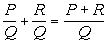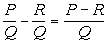Step 1: Combine the numerators together.   Step 2: Put the sum or difference found in step 1 over the common denominator.   Step 3: Reduce to lowest terms as shown in Tutorial 8: Simplifying Rational Expressions.

 Why do we have to have a common denominator when we add or subtract rational expressions?????

 Good question.  The denominator indicates what type of fraction that you have and the numerator is counting up how many of that type you have.  You can only directly combine fractions that are of the same type (have the same denominator).  For example if 2 was my denominator, I would be counting up how many halves I had.  If 3 was my denominator, I would be counting up how many thirds I had.  But I would not be able to add a fraction with a denominator of 2 directly with a fraction that had a denominator of 3 because they are not the same type of fraction.  I would have to find a common denominator first, which we will cover after the next two examples.Example 1:  Add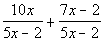.

 Since the two denominators are the same, we can go right into adding these two rational expressions.

 Step 1: Combine the numerators together AND Step 2: Put the sum or difference found in step 1 over the common denominator.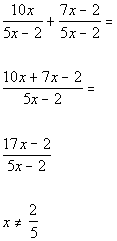*Common denominator of 5x - 2     *Combine the numerators *Write over common denominator         *Excluded values of the original den.

 Step 3: Reduce to lowest terms.

 Note that neither the numerator nor the denominator will factor.  The rational expression is as simplified as it gets. Also note that the value that would be excluded from the domain is 2/5.  This is the value that makes the original denominator equal to 0.Example 2:  Subtract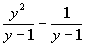.

 Since the two denominators are the same, we can go right into subtracting these two rational expressions.

 Step 1: Combine the numerators together AND Step 2: Put the sum or difference found in step 1 over the common denominator.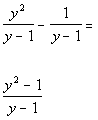*Common denominator of y - 1   *Combine the numerators *Write over common denominator

 Step 3: Reduce to lowest terms.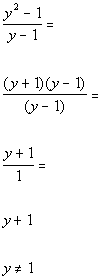*Factor the num. *Simplify by div. out the common factor of (y - 1)             *Excluded values of the original den.

 Note that the value that would be excluded from the domain is 1.  This is the value that makes the original denominator equal to 0.

 Least Common Denominator (LCD)

Step 1: Factor all the denominators

 If you need a review on factoring, feel free to go back to Tutorial 7: Factoring Polynomials.

Step 2: The LCD is the list of all the DIFFERENT factors in the denominators raised to the highest power that there is of each factor.

 Adding and Subtracting Rational Expressions  Without a Common Denominator

 Step 1: Find the LCD as shown above if needed.

 Step 2: Write equivalent fractions using the LCD if needed.

 If we multiply the numerator and denominator by the exact same expression it is the same as multiplying it by the number 1.  If that is the case,  we will have equivalent expressions when we do this.  Now the question is WHAT do we multiply top and bottom by to get what we want?  We need to have the LCD, so you look to see what factor(s) are missing from the original denominator that is in the LCD.  If there are any missing factors then that is what you need to multiply the numerator AND denominator by.

 Step 3: Combine the rational expressions as shown above.

 Step 4: Reduce to lowest terms as shown in Tutorial 8: Simplifying Rational Expressions.Example 3:  Add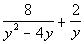.

 Step 1: Find the LCD as shown above if needed.

 The first denominator has the following two factors: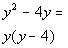*Factor the GCF

 The second denominator has the following factor: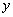Putting all the different factors together and using the highest exponent, we get the following LCD: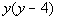Step 2: Write equivalent fractions using the LCD if needed.

 Since the first rational expression already has the LCD, we do not need to change this fraction.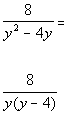*Rewriting denominator in factored form

 Rewriting the second expression with the LCD: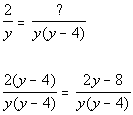*Missing the factor of (y - 4) in the den. *Mult. top and bottom by (y - 4)

 Step 3: Combine the rational expressions as shown above.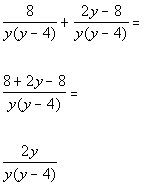*Combine the numerators *Write over common denominator

 Step 4: Reduce to lowest terms.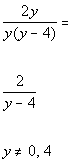*Simplify by div. out the common factor of y     *Excluded values of the original den.

 Note that the values that would be excluded from the domain are 0 and 4.  These are the values that make the original denominator equal to 0.Example 4:  Add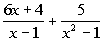.

 Step 1: Find the LCD as shown above if needed.

 The first denominator has the following factor: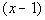The second denominator has the following two factors: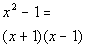*Factor the difference of squares

 Putting all the different factors together and using the highest exponent, we get the following LCD: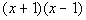Step 2: Write equivalent fractions using the LCD if needed.

 Rewriting the first expression with the LCD: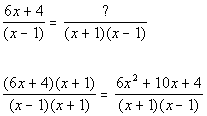*Missing the factor of (x + 1) in the den. *Mult. top and bottom by (x + 1)

 Since the second rational expression already has the LCD, we do not need to change this fraction.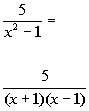*Rewriting denominator in factored form

 Step 3: Combine the rational expressions as shown above.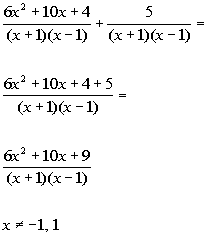*Combine the numerators *Write over common denominator               *Excluded values of the original den.

 Step 4: Reduce to lowest terms.

 This rational expression cannot be simplified down any farther.

 Also note that the values that would be excluded from the domain are -1 and 1.  These are the values that make the original denominator equal to 0.Example 5:  Subtract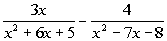.

 Step 1: Find the LCD as shown above if needed.

 The first denominator has the following two factors: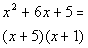*Factor the trinomial

 The second denominator has the following two factors: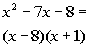*Factor the trinomial

 Putting all the different factors together and using the highest exponent, we get the following LCD: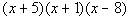Step 2: Write equivalent fractions using the LCD if needed.

 Rewriting the first expression with the LCD: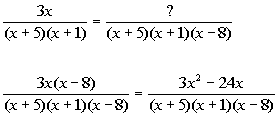*Missing the factor of (x - 8) in the den. *Mult. top and bottom by (x - 8)

 Rewriting the second expression with the LCD: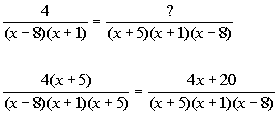*Missing the factor of (x + 5) in the den. *Mult. top and bottom by (x + 5)

 Step 3: Combine the rational expressions as shown above.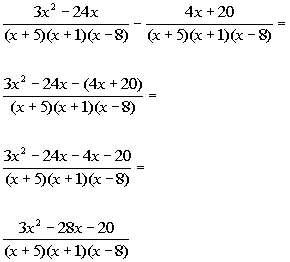*Combine the numerators *Write over common denominator   *Distribute the minus sign through the (   )

 Step 4: Reduce to lowest terms.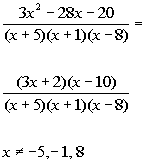*Factor the num. *No common factors to divide out   *Excluded values of the original den.

 Note that the values that would be excluded from the domain are -5,  -1 and 8.  These are the values that make the original denominator equal to 0.Practice Problems

 These are practice problems to help bring you to the next level.  It will allow you to check and see if you have an understanding of these types of problems. Math works just like anything else, if you want to get good at it, then you need to practice it.  Even the best athletes and musicians had help along the way and lots of practice, practice, practice, to get good at their sport or instrument.  In fact there is no such thing as too much practice. To get the most out of these, you should work the problem out on your own and then check your answer by clicking on the link for the answer/discussion for that  problem.  At the link you will find the answer as well as any steps that went into finding that answer.Practice Problems 1a - 1b: Perform the indicated operation.

 1a.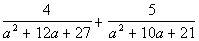(answer/discussion to 1a) 1b.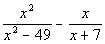(answer/discussion to 1b)Need Extra Help on these Topics?

The following are webpages that can assist you in the topics that were covered on this page: